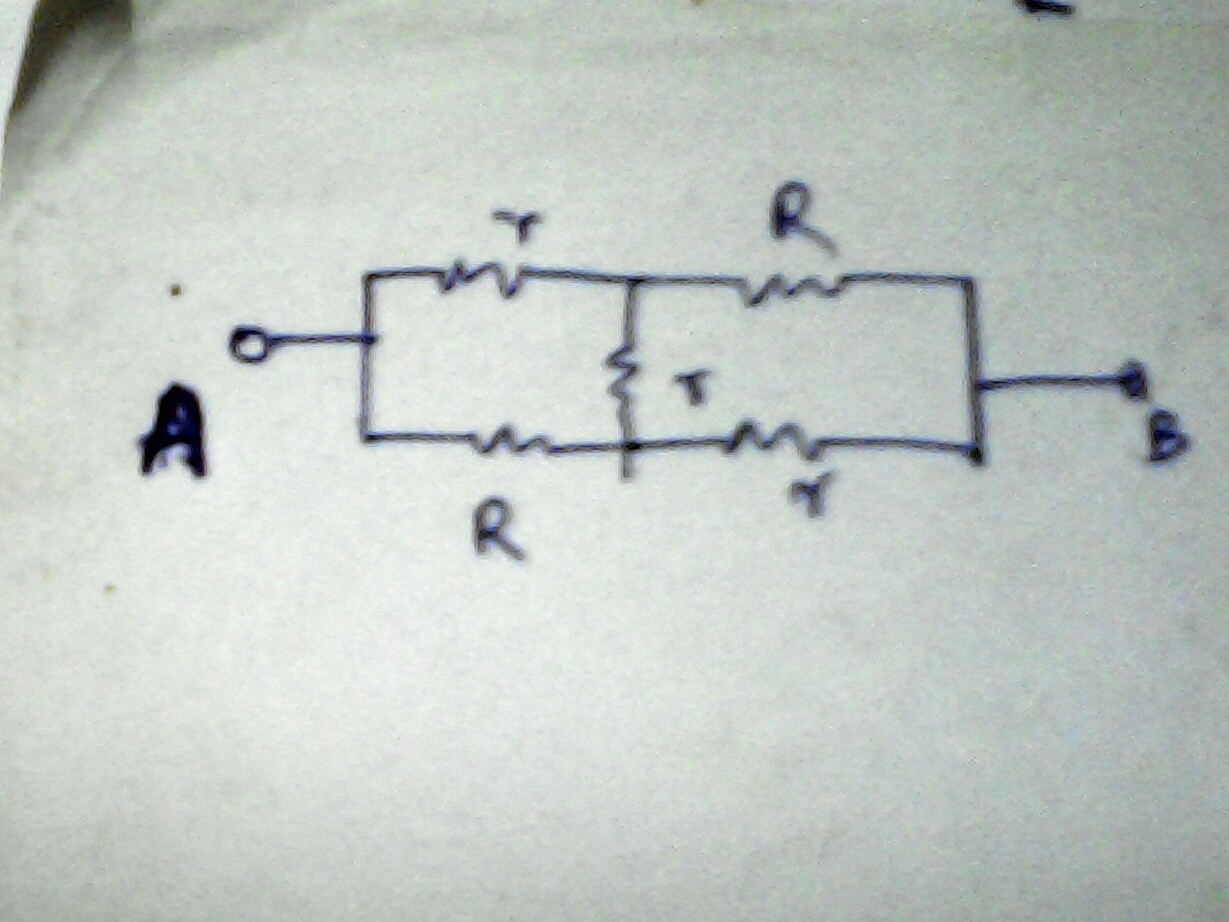# Resisting resistanceIn the given diagram, if the equivalent resistance between the points A and B can be written as $R' = \frac{aR + br}{c + \frac{dR}r}$. What is $a+b+c+d$?

Note that the expression is in its simplest form.

×